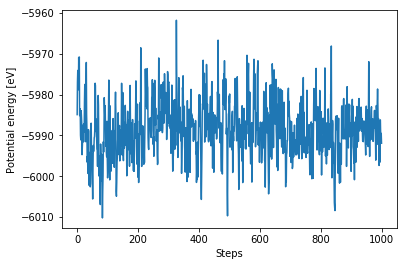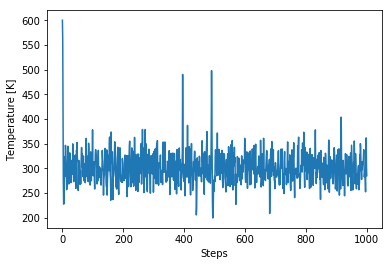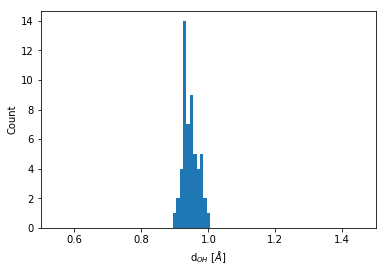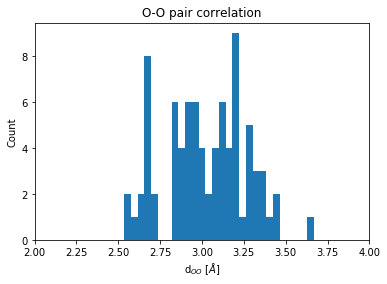# Molecular dynamics simulations of bulk water¶

In this example, we show how to perform molecular dynamics of bulk water using the popular interatomic TIP3P potential (W. L. Jorgensen et. al.) and LAMMPS.

In :
import numpy as np
%matplotlib inline
import matplotlib.pylab as plt
from pyiron.project import Project
import ase.units as units

In :
pr = Project("tip3p_water")


## Creating the initial structure¶

We will setup a cubic simulation box consisting of 27 water molecules density density is 1 g/cm$^3$. The target density is achieved by determining the required size of the simulation cell and repeating it in all three spatial dimensions

In :
density = 1.0e-24  # g/A^3
n_mols = 27
mol_mass_water = 18.015 # g/mol

# Determining the supercell size size
mass = mol_mass_water * n_mols / units.mol  # g
vol_h2o = mass / density # in A^3
a = vol_h2o ** (1./3.) # A

# Constructing the unitcell
n = int(round(n_mols ** (1. / 3.)))

dx = 0.7
r_O = [0, 0, 0]
r_H1 = [dx, dx, 0]
r_H2 = [-dx, dx, 0]
unit_cell = (a / n) * np.eye(3)
water = pr.create_atoms(elements=['H', 'H', 'O'],
positions=[r_H1, r_H2, r_O],
cell=unit_cell)
water.set_repeat([n, n, n])
water.plot3d()

In :
water.get_chemical_formula()

Out:
'H54O27'

## Equilibrate water structure¶

The initial water structure is obviously a poor starting point and requires equilibration (Due to the highly artificial structure a MD simulation with a standard time step of 1fs shows poor convergence). Molecular dynamics using a time step that is two orders of magnitude smaller allows us to generate an equilibrated water structure. We use the NVT ensemble for this calculation:

In :
job_name = "water_slow"
ham = pr.create_job("Lammps", job_name)
ham.structure = water
# Listing available potentials for this structure
ham.list_potentials()

Out:
['H2O_tip3p', 'H2O_tip3p_slab']

We will use the H2O_tip3p potential. The H2O_tip3p_slab should be used if you want to switch off the periodic boundary conditions along the z-axis

In :
ham.potential = 'H2O_tip3p'
ham.calc_md(temperature=300,
n_ionic_steps=1e4,
time_step=0.01)
ham.run()

In :
view = ham.animate_structure()
view


## Full equilibration¶

At the end of this simulation, we have obtained a structure that approximately resembles water. Now we increase the time step to get a reasonably equilibrated structure

In :
# Get the final structure from the previous simulation
struct = ham.get_structure(iteration_step=-1)
job_name = "water_fast"
ham_eq = pr.create_job("Lammps", job_name)
ham_eq.structure = struct
ham_eq.potential = 'H2O_tip3p'
ham_eq.calc_md(temperature=300,
n_ionic_steps=1e4,
n_print=10,
dt=1)
ham_eq.run()

In :
view = ham_eq.animate_structure()
view


We can now plot the trajectories

In :
plt.plot(ham_eq["output/generic/energy_pot"])
plt.xlabel("Steps")
plt.ylabel("Potential energy [eV]");In :
plt.plot(ham_eq["output/generic/temperatures"])
plt.xlabel("Steps")
plt.ylabel("Temperature [K]");## Structure analysis¶

We will now use the get_neighbors() function to determine structural properties from the computed trajectories. We take advantage of the fact that the TIP3P water model is a rigid water model which means the neighbors of each molecule never change. Therefore they need to be indexed only once

In :
final_struct = ham_eq.get_structure(iteration_step=-1)

# Get the indices based on species
O_indices = final_struct.select_index("O")
H_indices = final_struct.select_index("H")

# Getting only the first two neighbors
neighbors = final_struct.get_neighbors(num_neighbors=2)


### Distribution of the O-H bond length¶

Every O atom has two H atoms as immediate neighbors. The distribution of this bond length is obtained by:

In :
bins = np.linspace(0.5, 1.5, 100)
plt.hist(neighbors.distances[O_indices].flatten(), bins=bins)
plt.xlim(0.5, 1.5)
plt.xlabel("d$_{OH}$ [$\AA$]")
plt.ylabel("Count");### Distribution of the O-O bond lengths¶

We need to extend the analysis to go beyond nearest neighbors. We do this by controlling the cutoff distance

In :
neighbors = final_struct.get_neighbors(cutoff=8)

In :
neigh_indices = np.hstack(neighbors.indices[O_indices])
neigh_distances = np.hstack(neighbors.distances[O_indices])


One is often intended in an element specific pair correlation function. To obtain for example, the O-O coordination function, we do the following:

In :
# Getting the neighboring Oxyhen indices
O_neigh_indices  = np.in1d(neigh_indices, O_indices)
O_neigh_distances = neigh_distances[O_neigh_indices]

In :
bins = np.linspace(1, 5, 100)
count = plt.hist(O_neigh_distances, bins=bins)
plt.xlim(2, 4)
plt.title("O-O pair correlation")
plt.xlabel("d$_{OO}$ [$\AA$]")
plt.ylabel("Count");In [ ]: